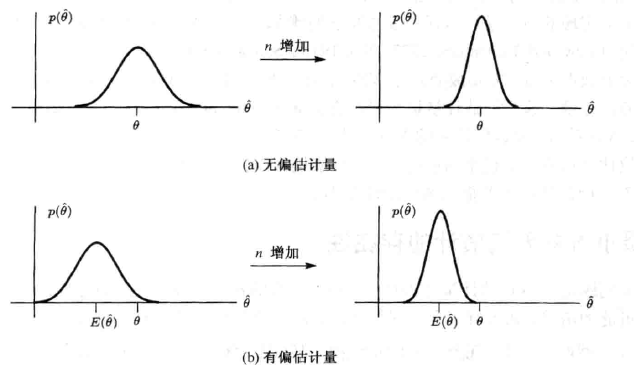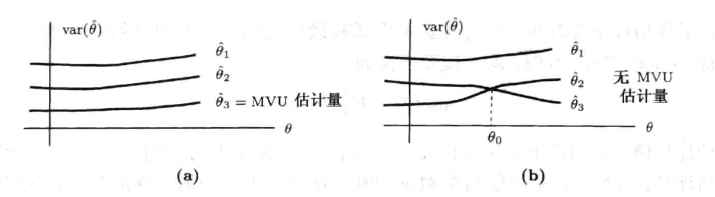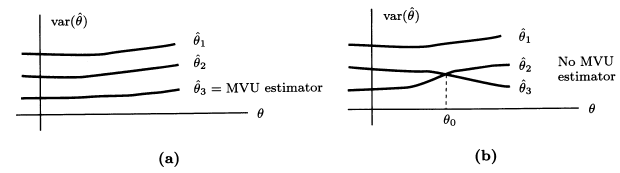• 数理统计8：点估计的有效性、一致最小方差无偏估计(UMVUE)、零无偏估计
千次阅读
2021-03-13 16:09:22

在之前的学习中，主要基于充分统计量给出点估计，并且注重于点估计的无偏性与相合性。然而，仅有这两个性质是不足的，无偏性只能保证统计量的均值与待估参数一致，却无法控制统计量可能偏离待估参数的程度；相合性只能在大样本下保证统计量到均值的收敛性，但却对小样本情形束手无策。今天我们将注重于统计量的有效性，即无偏统计量的抽样分布的方差。由于本系列为我独自完成的，缺少审阅，如果有任何错误，欢迎在评论区中指出，谢谢！

目录

Part 1：一致最小方差无偏估计

首先考虑这样的问题：如何刻画一个统计量的有效程度？注意到，一个统计量的取值既可能高于待估参数，亦可能低于待估参数，要综合考虑统计量对待估参数误差，需要用平方均衡这种双向偏差，因此，提出均方误差的概念：若$$\hat g(\boldsymbol{X})$$是$$g(\theta)$$的估计量，则$$\hat g(\boldsymbol{X})$$的均方误差定义为

$\mathrm{MSE}(\hat g(\boldsymbol{X}))= \mathbb{E}[\hat g(\boldsymbol{X})-g(\theta)]^2.$

对于确定的统计量$$\hat g(\boldsymbol{X})$$而言，$$\mathrm{MSE}(\hat g(\boldsymbol{X}))$$是$$\theta$$的函数。显然，一个统计量的均方误差越小，它就越在待估参数真值附近环绕，由此，用统计量的一次观测值作为待估参数的估计就有着越大的把握。

如果对于$$g(\theta)$$的两个估计量$$\hat g_1(\boldsymbol{X})$$和$$\hat g_2(\boldsymbol{X})$$，恒有$$\mathrm{MSE}(\hat g_1(\boldsymbol{X}))\le \mathrm{MSE}(\hat g_2(\boldsymbol{X}))$$，且严格不等号至少在某个$$\theta$$处成立，就称$$\hat g_1(\boldsymbol{X})$$在均方误差准则下优于$$\hat g_2(\boldsymbol{X})$$。如果我们能找到均方误差最小的统计量$$\hat g(\boldsymbol{X})$$，就相当于找到了均方误差准则下的最优统计量。

不过，均方误差是$$\theta$$的函数，这就导致了某些统计量在$$\theta=\theta_1$$时均方误差小，在$$\theta=\theta_2$$时均方误差大，一致最小均方误差估计量便不存在，需要增加约束条件，找到更可能存在的“最优”。

基于此，我们提出一致最小方差无偏估计(UMVUE)的概念，它将$$g(\theta)$$的估计量限制在了无偏估计之中，这使得UMVUE的存在可能性得以提高。并且，由于$$\mathbb{E}(\hat g(\boldsymbol{X}))=g(\theta)$$，所以

$\mathrm{MSE}(\hat g(\boldsymbol{X}))=\mathbb{E}(\hat g(\boldsymbol{X})-g(\theta))^2=\mathbb{E}[\hat g(\boldsymbol{X})-\mathbb{E}(\hat g(\boldsymbol{X}))]^2=\mathbb{D}(\hat g(\boldsymbol{X})),$

即无偏估计的均方误差就是无偏估计的方差。

不过首先要提出的是，UMVUE往往比一致最小均方误差估计量更容易存在，但依然不是所有参数都存在UMVUE的，并且，甚至可能有的参数根本不存在无偏估计。

以下是一个典型的不存在无偏估计的例子：$$X\sim B(n,p)$$，参数$$g(p)=1/p$$的无偏估计不存在。书上给出的证明过程如下：

首先，无偏估计不依赖于样本容量，故假设$$n=1$$。若$$g(p)$$有无偏估计$$\hat g(X)$$，则由于$$X=0,1,\cdots,n$$，故$$\hat g(X)$$的取值只可能是$$a_0,a_1,\cdots,a_n$$，可以写出其期望为

$\mathbb{E}(\hat g(X))=\sum_{j=0}^n a_jC_n^jp^j(1-p)^{n-j},$

当$$\hat g(X)$$为无偏估计时，成立以下等式：

$\sum_{j=0}^na_jC_n^jp^j(1-p)^{n-j}=\frac{1}{p},$

即对于某个多项式$$f\in\mathcal P_{n+1}(\mathbb{R})$$，有

$f(p)=0,\quad \forall p\in(0,1).$

显然，要使上式恒成立，除非$$f$$为零多项式。但$$f$$并不是零多项式，这意味着$$f(p)=0$$只会在至多$$n+1$$个点处成立，这与无偏性要求矛盾。因此，$$g(p)=1/p$$不存在无偏估计。

我们将存在无偏估计的待估参数称为可估参数，因此UMVUE仅对可估参数作讨论。我们的任务，就是用一定的方法，找到可估参数的UMVUE。

Part 2：改进无偏估计量

无偏估计量有许多，比如正态分布$$N(\mu,\sigma^2)$$中，$$\mu$$的无偏估计就有$$X_1,2X_2-X_1,\bar X$$等。充分统计量的条件期望法是改进无偏估计量的一个典型方式，它基于如下的定理：设$$T=T(\boldsymbol{X})$$是一个充分统计量，$$\hat g(\boldsymbol{X})$$是$$g(\theta)$$的一个普通无偏估计量，则$$h(T)=\mathbb{E}[\hat g(\boldsymbol{X})|T]$$是$$g(\theta)$$的无偏估计，且

$\mathbb{D}(h(T))\le \mathbb{D}(\hat g(\boldsymbol{X})),\quad \forall \theta\in\Theta.$

等号成立当且仅当$$\hat g(\boldsymbol{X})=h(T)$$是均方条件下成立的。它的证明不是很有必要掌握，权当了解。

因为$$T$$是$$g(\theta)$$的充分统计量，故$$\mathbb{E}(\hat g(\boldsymbol{X})|T)$$与待估参数$$g(\theta)$$无关，可以作为统计量，即

$h(T)=\mathbb{E}(\hat g(\boldsymbol{X})|T)$

是合理定义的统计量。下证其无偏性，由全期望公式，有

$\mathbb{E}(h(T))=\mathbb{E}[\mathbb{E}(\hat g(\boldsymbol{X})|T)]=\mathbb{E}(\hat g(\boldsymbol{X}))=g(\theta).$

最后证明其比$$\hat g(\boldsymbol{X})$$更有效，利用一个常用的拆分技巧，得到

\begin{aligned} \mathbb{D}(\hat g(\boldsymbol{X}))&=\mathbb{D}[\hat g(\boldsymbol{X})-h(T)+h(T)]\\ &=\mathbb{D}(h(T))+\mathbb{D}(\hat g(\boldsymbol{X})-h(T))+2\mathrm{Cov}(h(T),\hat g(\boldsymbol{X})-h(T)), \end{aligned}

然后证明交叉项为0，这里需要再次用到全期望公式，有

\begin{aligned} &\quad \mathrm{Cov}(h(T),\hat g(\boldsymbol{X})-h(T))\\ &=\mathbb{E}[h(T)-g(\theta)][\hat g(\boldsymbol{X})-h(T)]\\ &=\mathbb{E}[\mathbb{E}[(h(T)-g(\theta))(\hat g(\boldsymbol{X})-h(T))|T]]\\ &=\mathbb{E}[(h(T)-g(\theta))(\mathbb{E}(\hat g(\boldsymbol{X})|T)-h(T)]\\ &=0. \end{aligned}

最后的等号是因为$$h(T)=\mathbb{E}(\hat g(\boldsymbol{X})|T)$$，于是代回就得到

$\mathbb{D}(\hat g(\boldsymbol{X}))=\mathbb{D}(h(T))+\mathbb{D}(\hat g(\boldsymbol{X})-h(T))\ge \mathbb{D}(h(T)).$

等号成立当且仅当$$\mathbb{D}(\hat g(\boldsymbol{X})-h(T))^2=\mathbb{E}(\hat g(\boldsymbol{X})-h(T))^2=0$$。

这个定理的重要意义在于，如果给定的无偏估计量不是充分统计量的函数，则可以通过条件期望法，将其转变成一个充分统计量的函数作为新的统计量，并且新的统计量总是更有效的。此外，这也对我们寻找UMVUE提出启示：UMVUE一定是充分统计量的函数。如果不然，则可以通过对充分统计量求期望，得到一个更有效的统计量。

不过，改进后的充分统计量函数，尽管是更为有效的参数估计，但却并不一定是UMVUE，下面的定理将给出一个验证点估计是否为UMVUE的方法。

Part 3：零无偏估计法

零无偏估计法是用于判断某个估计量是否为UMVUE的方法，为此，首先要提出什么是零无偏估计。顾名思义，零无偏估计即零的无偏估计量，对某个统计量$$l(\boldsymbol{X})$$，如果有$$\mathbb{E}(l(\boldsymbol{X}))=0$$，则称$$l(\boldsymbol{X})$$是一个零无偏估计量；如果统计量$$T$$是待估参数$$g(\theta)$$的充分统计量，且$$\mathbb{E}(h(T))=0$$，则$$h(T)$$也称为$$g(\theta)$$的零无偏估计量。

零无偏估计法的思想、证明过程都与上述的充分统计量条件期望法类似。如果$$\hat g(\boldsymbol{X})$$是UMVUE，则对于任意其他无偏估计$$\hat g_1(\boldsymbol{X})$$，都可以视为$$\hat g_1(\boldsymbol{X})=\hat g(\boldsymbol{X})+l(\boldsymbol{X})$$，显然这里$$\mathbb{E}(l(\boldsymbol{X}))=0$$，要使$$\hat g_1(\boldsymbol{X})$$的方差大于$$\hat g(\boldsymbol{X})$$，可以进行拆分，即

$\mathbb{D}(\hat g_1(\boldsymbol{X}))=\mathbb{D}(\hat g(\boldsymbol{X}))+\mathbb{D}(l(\boldsymbol{X}))+2\mathrm{Cov}(\hat g(\boldsymbol{X}),l(\boldsymbol{X})).$

如果最后的协方差项为0，则必有$$\mathbb{D}(\hat g_1(\boldsymbol{X}))\ge \mathbb{D}(\hat g(\boldsymbol{X}))$$。综合以上讨论，给出零无偏估计法验证UMVUE的方式。

设$$\hat g(\boldsymbol{X})$$是$$g(\theta)$$的一个无偏估计，$$\mathbb{D}(\hat g(\boldsymbol{X})) $\mathrm{Cov}(\hat g(\boldsymbol{X}),l(\boldsymbol{X}))=0,$ 则\(\hat g(\boldsymbol{X})$$是$$g(\theta)$$的UMVUE。

如果$$\hat g(\boldsymbol{X})$$满足与任何零无偏估计无关，则它是UMVUE，这是一个充分条件。但反之，它也是一个必要条件，即UMVUE必定与任何零无偏估计量无关。

如果不然，设$$\mathrm{Cov}(\hat g(\boldsymbol{X}),l(\boldsymbol{X}))=b(\theta)\ne 0$$，则可以假设$$\mathbb{D}(l(\boldsymbol{X}))=a^2(\theta)>0$$。现在固定$$\theta=\theta_0$$为常数，并设$$a(\theta_0)=a,b(\theta_0)=b$$，只要

$\mathbb{D}(l(\boldsymbol{X}))+2\mathrm{Cov}(\hat g(\boldsymbol{X}),l(\boldsymbol{X}))=a^2(\theta_0)+2b(\theta_0)=a^2+2b<0,$

就能找到一个在$$\theta=\theta_0$$处，比$$\hat g(\boldsymbol{X})$$方差更小的无偏估计，那么$$\hat g(\boldsymbol{X})$$就不是UMVUE。注意到，如果$$l(\boldsymbol{X})$$是零无偏估计，则$$\forall k\ne 0$$，$$kl(\boldsymbol{X})$$也是零无偏估计，就有

$\mathbb{D}(kl(\boldsymbol{X}))+2\mathrm{Cov}(\hat g(\boldsymbol{X}),kl(\boldsymbol{X}))=k^2a^2+2bk,$

取$$k$$值为

$\left\{\begin{array}l -\frac{2b}{a}0; \\ 0 就使得$$k^2a^2+2bk<0$$成立，于是 \[\hat g_1(\boldsymbol{X})\xlongequal{def}\hat g(\boldsymbol{X})+kl(\boldsymbol{X})$

是$$g(\theta)$$的无偏估计量，且当$$\theta=\theta_0$$时$$\mathbb{D}(\hat g_1(\boldsymbol{X})) 有了这个方法，我们可以验证一些常用的充分统计量是UMVUE了。现以正态分布\(N(\mu,\sigma^2)$$的充分统计量$$(\bar X,S^2)$$为例，它们是否是UMVUE呢？如果直接验证会稍显繁琐，对零无偏估计法稍加修改可以得到以下的推论：

如果$$T$$是充分统计量且$$h(T)$$是$$g(\theta)$$的一个无偏估计，对任何$$\theta\in\Theta$$与一切零无偏估计量$$\delta (T)$$都有

$\mathrm{Cov}(h(T),\delta(T))=\mathbb{E}(h(T)\delta(T))=0,$

则$$h(T)$$是UMVUE。

这里只是将样本的函数改成了充分统计量的函数，以上证明过程是依然适用的。并且，由于UMVUE一定是充分统计量的函数，因此这个推论会更有应用意义。

对于正态分布而言，充分统计量可以视为

$T_1=\frac{1}{n}\sum_{j=1}^n X_j,\quad T_2=\sum_{j=1}^n (X_j-\bar X)^2,\\ T_1\sim N\left(\mu,\frac{\sigma^2}{n}\right),\quad \frac{T_2}{\sigma^2}\sim\chi^2(n-1)\Rightarrow T_2\sim\Gamma\left(\frac{n-1}{2},\frac{1}{2\sigma^2} \right).$

由于$$T_1,T_2$$独立，所以其联合密度函数容易写出，有

$f_1(t_1)=\frac{\sqrt{n}}{\sqrt{2\pi \sigma^2}}\exp\left\{-\frac{n(t_1-\mu)^2}{2\sigma^2} \right\},\\ f_2(t_2)=\frac{1}{2^{\frac{n-1}{2}}\Gamma(\frac{n-1}{2})\sigma^{n-1}}t_2^{\frac{n-1}{2}-1}e^{-\frac{t_2}{2\sigma^2}},\\ f(t_1,t_2)=\frac{C}{\sigma^n}t_2^{\frac{n-1}{2}-1}\exp\left\{-\frac{n(t_1-\mu)^2+t_2}{2\sigma^2} \right\}.$

如果$$\delta(t_1,t_2)$$是零均值的，则有

$\mathbb{E}(\delta(t_1,t_2))=\frac{C}{\sigma^n}\int_{-\infty}^\infty \delta(t_1,t_2)\cdot t_2^{\frac{n-1}{2}-1}\exp\left\{-\frac{n(t_1-\mu)^2+t_2}{2\sigma^2} \right\}\mathrm{d}t_1\mathrm{d}t_2=0.$

令$$\exp$$部分为$$H(\mu,\sigma^2)$$，也就是我们获得了这个关键的等式(它是证明的核心)：

$\int_{-\infty}^\infty\delta(t_1,t_2)\cdot t_2^{\frac{n-1}{2}-1}H(\mu,\sigma^2)\mathrm{d}t_1\mathrm{d}t_2=0$

要验证$$\mathbb{E}(t_1,\delta(t_1,t_2))$$与$$\mathbb{E}(t_2,\delta(t_1,t_2))$$是否为0，先从第一个入手，写出其表达式为

$\mathbb{E}(t_1,\delta(t_1,t_2))=\frac{C}{\sigma^n}\int_{-\infty}^{\infty}\delta(t_1,t_2)t_1t_2^{\frac{n-1}{2}-1}H(\mu,\sigma^2)\mathrm{d}t_1\mathrm{d}t_2.$

要验证$$\mathbb{E}(t_1,\delta(t_1,t_2))=0$$，实际上就是验证

$\int_{-\infty}^\infty \delta(t_1,t_2)t_1t_2^{\frac{n-1}{2}-1}H(\mu,\sigma^2)\mathrm{d}t_1\mathrm{d}t_2\stackrel{?}=0.$

不要被这个庞然大物吓到，事实上我们唯一的条件只有$$\mathbb{E}(\delta(t_1,t_2))=0$$所对应的等式，注意到上面的等式无论$$\mu,\sigma$$的真值是多少都应该成立，所以是$$\mu,\sigma$$的二元函数，我们能做的事也很有限——对参数求导。这里涉及到了求导与积分是否可交换的问题，我们姑且不考虑，默认视为可交换即可，由于$$\sigma^2$$很复杂，所以对$$\mu$$求导即可。唯一含有$$\mu$$的项是积分号中间的$$\exp$$部分，其导数为

$H(\mu,\sigma^2)=\exp\left\{-\frac{n(t_1-\mu)^2+t_2}{2\sigma^2} \right\},\\ \frac{\partial H(\mu,\sigma^2)}{\partial \mu}=\frac{2n(t_1-\mu)}{\sigma^2}H(\mu,\sigma^2),$

于是就有

$\frac{\partial \mathbb{E}(\delta_1,\delta_2)}{\partial\mu}=\frac{C}{\sigma^n}\int_{-\infty}^\infty\delta(t_1,t_2)t_2^{\frac{n-1}{2}-1}\frac{2n(t_1-\mu)}{\sigma^2}H(\mu,\sigma^2)\mathrm{d}t_1\mathrm{d}t_2=0,$

将求导后得到的式子展开，提取出参数并代入上面的结果，就有

$\int_{-\infty}^\infty\delta(t_1,t_2)t_1t_2^{\frac{n-1}{2}-1}H(\mu,\sigma)\mathrm{d}t_1\mathrm{d}t_2=0,$

也就说明了$$\mathbb{E}(t_1,\delta(t_1,t_2))=0$$，由$$\delta(t_1,t_2)$$的任意性以及$$T_1$$的无偏性，可知$$\bar X$$是$$\mu$$的UMVUE。

下一步证明$$T_2/(n-1)$$是$$\sigma^2$$的UMVUE，也就是证明$$\mathbb{E}(t_2,\delta(t_1,t_2))=0$$，同样写出需要验证的等式为

$\int_{-\infty}^\infty\delta(t_1,t_2) t_2^{\frac{n-1}{2}}H(\mu,\sigma^2)\mathrm{d}t_1\mathrm{d}t_2\stackrel{?}=0.$

现在我们尝试将关键的等式对$$\sigma^2$$求导，同样，先计算$$H$$对$$\sigma^2$$的偏导，有

$H(\mu,\sigma^2)=\exp\left\{-\frac{n(t_1-\mu)^2+t_2}{2\sigma^2} \right\},\\ \frac{\partial H(\mu,\sigma^2)}{\partial \sigma^2}=\frac{n(t_1-\mu)^2+t_2}{2\sigma^4}H(\mu,\sigma^2),$

可以看到，这里除了出现已经确认代入能为0的常数项和$$t_1$$外，还多了$$t_1^2$$与$$t_2$$的项，$$t_2$$就是我们的目标，所以再处理一下$$t_1^2$$这一项。显然，对$$\mu$$求导一次能得到$$t_1$$的一次项，那么对$$\mu$$求二阶导，就能得到$$t_1^2$$项，所以

$\frac{\partial H(\mu,\sigma^2)}{\partial \mu}=\frac{2n(t_1-\mu)}{\sigma^2}H(\mu,\sigma^2),\\ \frac{\partial^2H(\mu,\sigma^2)}{\partial\mu^2}=H(\mu,\sigma^2)\left[\frac{4n^2(t_1-\mu)^2}{\sigma^4}-\frac{2n}{\sigma^2} \right].$

这样就出现了需要的$$t_1^2$$项，剩下的工作只有繁琐的代入计算而已，我们实际上已经完成了证明的主要步骤，因此$$S^2$$也是$$\sigma^2$$的UMVUE。

对于一元连续或离散情形下UMVUE的验证，难度要比二元情形下小得多，因此读者只要掌握了正态分布的零无偏估计法验证，理论上其他UMVUE的验证便不成问题。读者可以自己尝试其他UMVUE的验证。

今天，我们提出了UMVUE的概念，重点在于利用充分统计量改进普通的无偏估计，并且利用零无偏估计法验证某个充分统计量函数是否是UMVUE。但是，我们并没有给出寻找UMVUE的方法，如果依靠感觉没有方向地寻找再一个个验证是否为UMVUE，是十分繁琐且难以成功的。比如对于$$B(1,p)$$的参数估计$$g(p)=p(1-p)$$，如果用先猜想后验证的方法来寻找UMVUE，甚至没有入手点。

因此，明天我们将学习寻找UMVUE的方法，并介绍一个被我们忽略已久的概念——指数族。

更多相关内容
• 最小方差无偏估计 2.1 无偏估计量 ​ 无偏估计意味着估计量的平均值为未知参数的真值。如果 E[θ^]=θ,a<θ<b E[\hat{\theta}]=\theta, a<\theta<b E[θ^]=θ,a<θ<b 说明估计量θ^\hat{\theta}θ...

## 最小方差无偏估计

### 2.1 无偏估计量

​ 无偏估计意味着估计量的平均值为未知参数的真值。如果
E [ θ ^ ] = θ , a < θ < b E[\hat{\theta}]=\theta, a<\theta<b
说明估计量 θ ^ \hat{\theta} ​是无偏估计量。

​ 例：白色高斯噪声中DC电平的无偏估计量

观测值 x [ n ] = A + w [ n ]   n = 0 , 1 , . . . , N − 1 x[n]=A+w[n]\ n=0,1,...,N-1

观测值中的参数A即所需估计的DC电平。取合理的估计量为:
A ^ = 1 N ∑ n = 0 N − 1 x [ n ] \hat{A}=\frac{1}{N}\sum_{n=0}^{N-1}x[n]

估计量的期望为：
KaTeX parse error: No such environment: align at position 8: \begin{̲a̲l̲i̲g̲n̲}̲ E(\hat{A})&=E[…

​ 无偏估计量只是保证估计量的平均值为真值，相比于有偏估计量不存在系统误差。但是在多个无偏估计量的情况下，可以用多个估计量的平均获得更好的估计。
KaTeX parse error: No such environment: align at position 8: \begin{̲a̲l̲i̲g̲n̲}̲ \hat{\theta}&=…
​ 所以求平均的估计越多，其均值会收敛到真值，且估计量的方差越小。

​ 但是如果估计量为有偏估计 E ( θ ^ ) = θ + b ( θ ) E(\hat{\theta})=\theta+b(\theta) ，那么无论对多少估计量求平均，都不会收敛到真值。

估计量的偏差： b ( θ ) = E ( θ ^ ) − θ b(\theta)=E(\hat{\theta})-\theta​ 为了从多个无偏估计量中选择出最佳估计量，可以采用一些最佳准则。

均方误差(mean square error,MSE)表示的是估计量偏离真值的平方偏差的统计平均值，由估计量的方差和偏差导致的误差组成。
m s e ( θ ^ ) = E [ ( θ ^ − θ ) 2 ] = v a r ( θ ^ ) + [ E ( θ ^ ) − θ ] 2 = v a r ( θ ^ ) + b 2 ( θ ) mse(\hat{\theta}) =E[(\hat{\theta}-\theta)^2] \\ =var(\hat{\theta})+[E(\hat{\theta})-\theta]^2\\=var(\hat{\theta})+b^2(\theta)
​ 但是如果让均方误差对修正常数求导，寻找出最小均方误差时可以发现估计量修正常数与真值有关，说明估计量是不可实现的。因此
在实际运用中，需要放弃最小MSE估计。选择另外一种方法使得约束偏差为零，从而求出使得方差最小的估计量
，这样得到的估计量称为最小方差无偏估计(minimum variance unbiased,MVU)

​ MVU的存在性：MVU表明它的方差相对其它所有估计量的方差是最小的，如果存在一个估计量的方差小于MVU的，说明MVU不存在。针对下图，可以说a图存在MVU，而b图不存在MVU。### 求最小方差无偏估计量MVU

​ 即使MVU存在，一般也很难求出。下面给出了一些可能求出的方法：

1. 确定Cramer-Rao下限(CRLB)，然后检查估计量是否满足CRLB；
2. 应用Rao-Blackwell-lehmann-Scheffe(RBLS)定理；
3. 进一步限制估计不仅是无偏，而且还是线性的，在这些限制中找出MVU。

扩展到适量参数

​ 如果 θ = [ θ 1 , θ 2 , . . . , θ p ] \pmb\theta=[\theta_1,\theta_2,...,\theta_p] 是未知参数矢量，那么估计量 θ ^ = [ θ ^ 1 , θ ^ 2 , . . . , θ ^ p ] \hat{\pmb\theta}=[\hat\theta_1,\hat\theta_2,...,\hat\theta_p] ​如果满足
E ( θ ^ i ) = θ i E(\hat\theta_i)=\theta_i
则说明估计量为无偏估计，那么该估计量具有 E ( θ ^ ) = θ E(\hat{\pmb\theta})=\pmb\theta 。同样对于MVU，对该参数矢量中任意一个参数都有 v a r ( θ ^ i ) var(\hat\theta_i) 都是最小的。

展开全文数字信号处理
• 最小方差无偏估计[MVUE]

最小方差无偏估计

定义：
最小方差无偏估计MVUE是在无偏估计类中，使得均方误差MSE达到最小的估计量，即在均方误差最小意义下的最优估计。

求解：
设总体X的分布函数为 F ( x ; θ ) , θ ∈ Θ , ( X 1 , X 2 , ⋯   , X n ) T F(x;\theta ),\theta\in\varTheta,(X_1,X_2,\cdots ,X_n)^T 为其样本，若 T = ( X 1 , X 2 , ⋯   , X n ) T=(X_1,X_2,\cdots ,X_n) θ \theta 的充分完备统计量， θ ^ \hat{\theta} θ \theta 的一个无偏估计，则
θ ^ ∗ ≜ E ( θ ^ ∣ T ) \hat{\theta }^\ast \triangleq E(\hat{\theta }|T) θ \theta 的唯一的最小方差无偏估计。

举例：
泊松总体 P ( λ ) P(\lambda ) , X ‾ \overline{\Chi} 是参数 λ \lambda 的充分完备统计量且又是 λ \lambda 的一个无偏估计量，所以 E ( X ‾ \ X ‾ ) = X ‾ E(\overline{\Chi}\backslash\overline{\Chi})=\overline{\Chi} λ \lambda 的最小方差无偏估计。

正态总体

罗-克拉默不等式
有效估计

展开全文• - 确定好的估计量 - 建立数据的数学模型：一般由于数据固有的随机性，则选择它们的PDF来描述它，...- 最佳估计量的选择：估计量性能的评估（无偏性、有效性以及一致性）。常用的方法：期望验证无偏；CRLB（Cramer-R...

- 确定好的估计量

- 建立数据的数学模型：一般由于数据固有的随机性，则选择它们的PDF来描述它，即

- 运用PDF和相关约束条件来获取估计量：估计对象是一个确定性的参数，只是具体值未知。如果估计对象是一个随机变量，则需要引入估计对象的先验分布，利用贝叶斯方法进行估计。

- 最佳估计量的选择：估计量性能的评估（无偏性、有效性以及一致性）。常用的方法：期望验证无偏；CRLB（Cramer-Rao Lower Bound）和方差验证有效；一致性利用依概率收敛验证。

- 估计方法一——最小方差无偏估计(MVU)

- 无偏估计量：估计量的均值为未知参数的真值  即 。（注意：无偏估计量并不意味着它就是好的/最佳的估计量）

- 无偏性的另一个重要含义：当同一参数有多个估计量可用的请况  。 一个合理的解决方法，对这些估计组合求平均，从而获取更好的估计，即是。若干每个估计量是无偏，方差相同且互不相关，则有

- 有偏估计量：一般请况定义估计量的偏差为

- 最小方差准则：一个自然的准则是均方误差（MSE）

定义为

- MVUE是否存在：对所有的具有最小方差的无偏估计   例如下图（图片来源：Steven M. Kay《Fundamental of statistical signal processing estimation theory》）- 求MVUE：MVUE即使存在，可能也无法求取请况。有几种常用方法：1）利用CRLB，检查是否有某些参数估计量满足MVUE；2）应用RBLS(Rao-Blackwell-Lehman-Scheffe)定理；3）进一步限制估计量是无偏的，且还是线性的，然后在这些限制中找到MVU。这三个方法后面会逐一说明。

展开全文• 最小方差无偏估计 MVUE 文章目录最小方差无偏估计 MVUE1. 统计信号处理概述2. 信号处理举例2.1 问题描述2.2 处理方法2.3 处理原理2.3.1 系统建模2.3.2 处理分析2.3.2.1 方差2.3.2.2 均值2.3.3 更多次的加权平均3. ...
• - 回顾 ...前面一直在讲述估计量的有效性（CRLB，线性模型），而没有提到假如估计量的方差没有达到CRLB，即是有效估计量不存在，但能够求出MVU估计量（假定存在）仍然是一个重要的事（可参考文章中的图片...
• 描述了最小方差无偏估计的基本方法和原理，附加基本例题供参考。
• 在累积赔款额流量三角形的基础上,假设进展因子服从对数正态分布,得到未决赔款准备金的一致最小方差无偏估计及估计量的方差的一致最小方差无偏估计
• 1、问题描述：假设有一批样本{xi,i=1,2,3...,n−1,n{x_i,i=1,2,3...,n-1,n}xi​,i=1,2,3...,n−1,n}。 标准方差的公式为： S2=1n∑i=1n(xi−x‾)2S^{2} = \frac{1}{n}\sum_{i=1}^{n}(x_i - \overline{x})^{2}S2=n1​...
• 在准椭球等高分布下给出了生长曲线模型中tr（CV）的一致最小方差非负无偏估计存在及任一个非负次估计成为一致最小方差非负无偏估计的充要条件。
• 文章目录一、最小方差估计例子、线性最小方差估计三、其他最优估计1、极大验后估计2、极大似然估计四、总结 一、最小方差估计        前两篇文章对最优估计中的最小二乘估计...矩阵 算法
• 样本均值和样本方差 　首先对于样本$x_1...x_n$来说，他们的均值为与方差分别为： 　$\bar{x} = \frac{1}{n}\sum\limits_{i=1}^{n}x_i$ ...　要证明样本方差无偏性，首先要计算样本均值的方差。 样本均值的方差...
• 卡尔曼滤波就是线性最小方差估计 你根据线性最小方差估计就可以推导出卡尔曼滤波那些东西。 下面是截图自我的notability的笔记 下面摘自：https://blog.csdn.net/victor_zy/article/details/82862904 ...
• 什么是无偏估计？？ 估计是用样本统计量（可以理解为随机抽样）来估计总体参数时的一种偏推断。 无偏估计的要求就是：估计出来的参数的数学期望等于被估计参数的真实值。 所以呢,可以看出：估计值也是一个变量，...
• 楼主，我发现你最近发了很多这...偏性是指，比如我们要估计某个参数theta,然后得到一个估计量theta_hat,如果theta_hat的数学期望等于未知参数theta,那么我们说theta_hat是theta的无偏估计。引用版主的例子：样本方...
• ϵ\epsilonϵ假定为白噪声，方差为σ2\sigma^2σ2，y,Xy,Xy,X已经中心化 最小二乘法的解为 β^=(XTX)XTy=X+y \hat{\beta} = (X^TX)X^Ty = X^{+}y β^​=(XTX)XTy=X+y 无偏估量性质 E(β^)=E(X+y)=E(X+(Xβ+ϵ))=E(β...算法
• 目录极大似然估计问题背景极大似然估计的计算方法参数估计的有性和无偏性 极大似然估计 问题背景 以高斯分布引出问题，高斯分布的重要性体现于： 1.根据中心极限定理，当样本量足够大的时候，任意分布的均值都...
• ## 无偏估计量

千次阅读 2019-08-14 11:34:28
目录 ...5如何理解无偏估计量？ 5.1 偏性 5.2有效性 5.3一致性 5.4 总结 参考百度百科：https://baike.baidu.com/item/%E6%97%A0%E5%81%8F%E4%BC%B0%E8%AE%A1%E9%87%8F/303853?fr=aladdin ...
• 定义：使样本事件发生概率最大的参数值，作为总体参数的估计值，就是极大似然估计。 比如箱子中有100个球，共两种颜色白和黑。已知白球和黑球的比例是1...无偏性的含义是：用样本估计的参数值的期望，等于真实值。 ...机器学习 算法
• - 引言 这个问题提出的原因：MVU估计量即使存在可能也无法求出。比如：有些情况下，可能并不知道数据的PDF；...若准最佳MVU估计量的方差可确定，且满足当前估计问题的需求，那么可认定它可用；若不可用，则...
• 文章目录偏性定义3.2.1(偏差与无偏估计)定义3.2.2(一致最小风险无偏估计, UMRUE)定义3.2.2'(一致最小方差无偏估计, UMVUE) 偏性 定义3.2.1(偏差与无偏估计) 定义3.2.2(一致最小风险无偏估计, UMRUE) ...数理统计
• 最佳线性无偏估计BLUE 1、定义：线性估计是参数估计最重要的一类，应用 广泛。...2、定理：如果量测信息的协方差矩阵是非奇异的，对于任意分布的量测 z 和参数 x，有线性最小方差估计为： x^BLUE=x...
• ## 估计、偏差 、方差

千次阅读 2019-07-03 09:49:43
在监督学习中，已知样本 ，要求拟合出一个模型（函数），其预测值与样本实际值的误差最小。 考虑到样本数据其实是采样，并不是真实值本身，假设真实模型（函数）是，则采样值，其中代表噪音，其均值为0，方差为。 ...
• 线性分布 通过具体因果关系的一组观察数据或者，建立线性模型的多变量分析方法。线性回归可分为一元线性回归和多元线性回归。下面将介绍一元线性回归模型。 当可控变量只有一个时，回归函数可表示为 (1) 则 ...
• 最近学习PCA，在求最大化方差 σ2=1P−1∑k=1P(vT(xk−μ))2−λ(∥v∥2−1)\sigma^{2} = \frac{1}{P-1} \sum_{k=1}^{P}(v^{T}(x_{k}-\mu ))^{2}-\lambda(\left \| v \right \|^{2}-...时遇到了无偏估计的问题——为...
•  在上一个主题中，也是一个与回归相关的，不过上一节更侧重于梯度这个概念，这一节更侧重于回归本身与偏差和方差的概念。  回归最简单的定义是，给出一个点集D，用一个函数去拟合这个点集，并且使得点集与拟合...
• 章 参数估计 在解决实际问题中，当确定了总体的分布族后，我们要从样本来推断总体的具体分布或感兴趣的总体特征数。例如，总体的数学期望和方差等。统计推断主要分为参数估计和假设检验，参数估计又分为点估计和...数理统计 参数估计 均方误差准则
• 卡方分布： 首先我们先把现代数学中的数理统计中的卡方分布已经烂大街的定义先放下来，我先回到卡方检验的诞生的之地。 在1900年，皮尔森发表了著名的关于卡方检验的文章，该文章被认为是现代统计学的基石之一。...
• 正态分布 and 最小二乘法 最小二乘法的过往 正态分布的前生 二者的联姻...